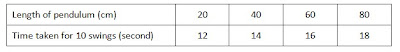## Monday 16 August 2010

### Success Science

Paper 2 – Structured Question

Diagram below show the pendulum experiment.The table below show the result of the investigation.1. State the hypothesis tested in the experiment.
Tip: Hypothesis is the relationship between the manipulated variable [what is changed] and the responding variable [what is observed].

2. State the variables involved in this experiment.
i. Manipulated variable
ii. Responding variable
iii. Constant variable
Tip: The manipulated variable and responding variable can be obtained from the table. Constant variable means that if it is changed, the readings of the experiment will be change.

3. Estimate the time taken for the pendulum to make ten complete swings when the length of pendulum is 100cm.
Tip: Student must check the time interval.

4. State the relationship between the times taken for ten complete swings with its length.
Tip: Student have to check the pattern if it is increasing or decreasing.

5. Based on table above, sketch a graph of the pendulum length against the period of the pendulum swing.
Tip: If kind of graph is not stated, student should draw a line graph. Please use ruler to join the points. Mark the points clearly with an “x” symbol.

Good Luck!

## Wednesday 11 August 2010

### Effect of Heat on Matter1. The three (3) states of matter are solids, liquids and gases.
2. Matter can change from one state to another when it gain heat or loses heat.
3. Heat is the energy that determines the movement of particles in matter. If the temperature of matter increases, the particles will get higher kinetic energy to move faster.

4. The changes in the state of matter which take place when substance gain heat are:
a) Melting (ice → water)
b) Boiling (water → steam) / Evaporation (water → water vapour)
c) Sublimation (mothballs → vapour, dry ice [solid carbon dioxide] → carbon dioxide gas)

5. The change in the state of matter which take place when substance loses heat are:
a) Condensation (water vapour → dew)
b) Freezing (water → ice)
c) Sublimation (iodine gas → iodine crystals)

6. Differences between boiling and evaporation:
Boiling
• Gas bubbles are produced
• Happens at boiling point of liquids
• Happens throughout the liquid
• A fast process

Evaporation
• Gas bubbles are not produced
• Happens at all temperatures
• Happens at surface of liquid
• A slow process